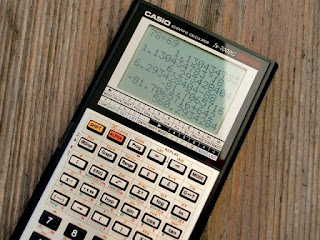## December 25, 2013

### FCC Technician Exam Question Of The Day (T5B11)

Q) What is the approximate amount of change, measured in decibels (dB), of a power increase from 20 watts to 200 watts?

A) 10 dB

NJ2X Notes:
You can use the following formula to calculate the ratio in decibels given two different power values.

dB = 10 * Log(Pa / Pb)

Using the figures in the question:
dB = 10 * Log (200 / 20)
dB = 10 * Log(10)
dB = 10 * 1
dB = 10Casio fx-7000G calculator